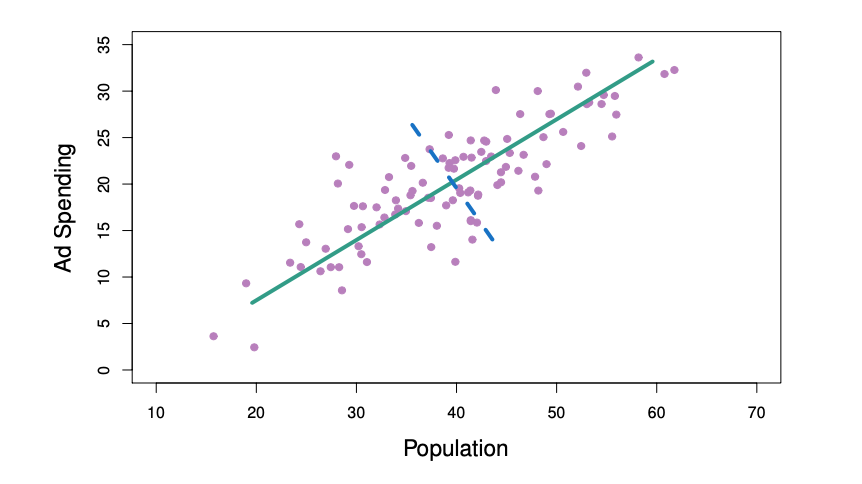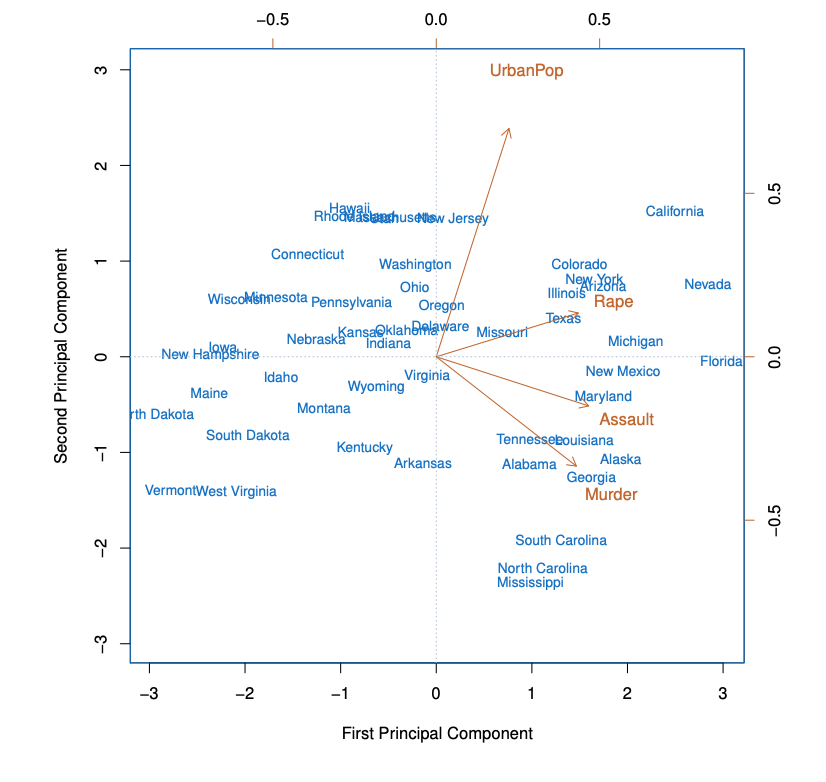## Unsupervised Learning

• Unsupervised vs Supervised Learning:

• Most of our focus has been on supervised learning methods such as regression and classification

• In that setting we observe both a set of features $$X_1, X_2, ..., X_p$$ for each object, as well as a response or outcome variable $$Y$$. The goal is then to predict Y using $$X_1, X_2, ..., X_p$$

• Here we instead focus on unsupervised learning, we where observe only the features $$X_1, X_2, ..., X_p$$. We are not interested in prediction, because we do not have an associated response variable Y

## The Goals of Unsupervised Learning

• The goal is to discover interesting things about the measurements: is there an informative way to visualize the data? Can we discover subgroups among the variables or among the observations?

• We discuss two methods:

• Principal components analysis, a tool used for data visualization or data pre-processing before supervised techniques are applied

• Clustering, a broad class of methods for discovering unknown subgroups in data

## The Challenge of Unsupervised Learning

• Unsupervised learning is more subjective than supervised learning, as there is no simple goal for the analysis, such as prediction of a response

• But techniques for unsupervised learning are of growing importance in a number of fields:

• Subgroups of breast cancer patients grouped by their gene expression measurements

• Groups of shoppers characterized by their browsing and purchase histories

• Movies grouped by the ratings assigned by movie viewers

• It is often easier to obtain unlabeled data — from a lab instrument or a computer — than labeled data, which can require human intervention

• For example it is difficult to automatically assess the overall sentiment of a movie review: is it favorable or not?

## Principal Components Analysis

• PCA produces a low-dimensional representation of a dataset. It finds a sequence of linear combinations of the variables that have maximal variance, and are mutually uncorrelated

• Apart from producing derived variables for use in supervised learning problems, PCA also serves as a tool for data visualization

• The first principal component of a set of features $$X_1, X_2, ..., X_p$$ is the normalized linear combination of the features

• $$Z_1 = \phi_{11}X_1 + \phi_{21}X_2 + ... + \phi_{p1}X_p$$

• that has the largest variance. By normalized, we mean that $$\sum_{j=1}^{p} \phi_{j1}^2= 1$$
• We refer to the elements $$\phi_{11}, ..., \phi_{p1}$$ as the loadings of the first principal component; together, the loadings make up the principal component loading vector $$\phi_1 = (\phi_{11} \phi_{21} ... \phi_{p1})^T$$

• We constrain the loadings so that their sum of squares is equal to one, since otherwise setting these elements to be arbitrarily large in absolute value could result in an arbitrarily large variance

• Example

• The population size (pop) and ad spending (ad) for 100 different cities are shown as purple circles. The green solid line indicates the first principal component direction, and the blue dashed line indicates the second principal component direction## Computation of Principal Components

• Suppose we have a $$n×p$$ data set $$X$$. Since we are only interested in variance, we assume that each of the variables in $$X$$ has been centered to have mean zero (that is, the column means of $$X$$ are zero)

• We then look for the linear combination of the sample feature values of the form

• $$z_{i1} = \phi_{11}x_{i1} + \phi_{21}x_{i2} + ... + \phi_{p1}x_{ip}$$

• For $$i=1,...,n$$ that has the largest sample variance, subject to the constraint that $$\sum_{j=1}^{p}\phi_{j1}^{2}=1$$
• Since each of the $$x_{ij}$$ has mean zero, then so does $$z_{i1}$$ (for any values of $$\phi_{j1}$$). Hench the sample variance of the $$z_{i1}$$ can be written as $$\frac{1}{n} \sum_{i=1}^{n}z_{i1}^2$$

• Plugging in (1) the first principal component loading vector solves the optimization problem

• $$\underset{\phi_{11},...,\phi_{p1}}{maximize} \frac{1}{n} \sum_{i=1}^{n} (\sum_{j=1}^{p} \phi_{j1}x_{ij})^2$$

• Subject to $$\sum_{j=1}^{p} \phi_{j1}^2 = 1$$
• This problem can be solved via a singular-value decomposition of the matrix $$X$$, a standard technique in linear algebra

• We refer to $$Z_1$$ as the first principal component, with realized values $$z_{11},...,z_{n1}$$

### Geometry of PCA

• The loading vector φ1 with elements $$\phi_{11}, \phi_{21},...,\phi_{p1}$$ defines a direction in feature space along which the data vary the most

• If we project the n data points $$x_1,...,x_n$$ onto this direction, the projected values are the principal component scores $$z_{11},...,z_{n1}$$ themselves

### PCA Continued

• The second principal component is the linear combination of $$X_1,...,X_p$$ that has maximal variance among all linear combinations that are uncorrelated with $$Z_1$$

• The second principal component scores $$z_{12},z{22},...,z_{n2}$$ take the form

• $$z_{i2} = \phi_{12}x_{i1} + \phi_{22}x_{i2} + ... + \phi_{p2}x_{ip}$$

• Where $$\phi_2$$ is the second principal component loading vector, with elements $$\phi_{12}, \phi_{22},...,\phi_{p2}$$
• It turns out that constraining $$Z_2$$ to be uncorrelated with $$Z_1$$ is equivalent to constraining the direction $$\phi_2$$ to be orthogonal (perpendicular) to the direction $$\phi_1$$. And so on

• The principal component directions $$\phi_1, \phi_2, \phi_3, . . .$$ are the ordered sequence of right singular vectors of the matrix $$\mathbf{X}$$, and the variances of the components are $$\frac{1}{n}$$ times the squares of the singular values. There are at most $$min(n − 1, p)$$ principal components

• Illustration

• USAarrests data: For each of the fifty states in the United States, the data set contains the number of arrests per 100, 000 residents for each of three crimes: Assault, Murder, and Rape. We also record UrbanPop (the percent of the population in each state living in urban areas)

• The principal component score vectors have length n = 50, and the principal component loading vectors have length p = 4

• PCA was performed after standardizing each variable to have mean zero and standard deviation one• The first two principal components for the USArrests data

• The blue state names represent the scores for the first two principal components

• The orange arrows indicate the first two principal component loading vectors (with axes on the top and right). For example, the loading for Rape on the first component is 0.54, and its loading on the second principal component 0.17 [the word Rape is centered at the point (0.54, 0.17)]

• This figure is known as a biplot, because it displays both the principal component scores and the principal component loadings